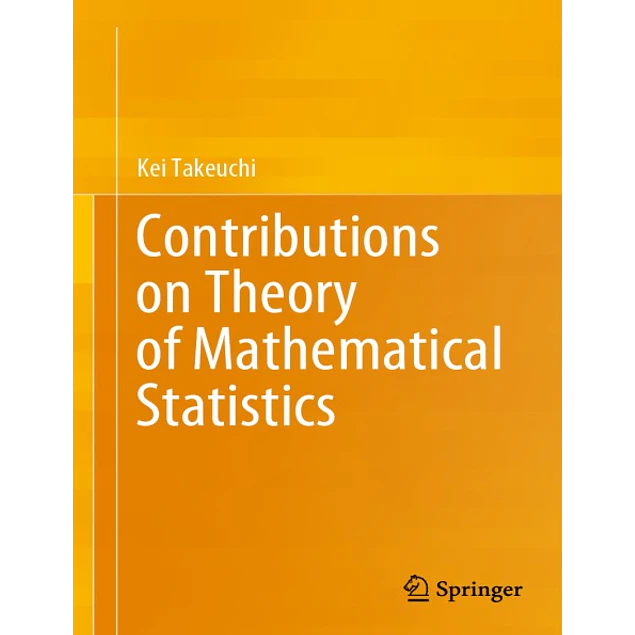## Contributions on Theory of Mathematical Statistics

20.00\$# Contributions on Theory of Mathematical Statistics

20.00\$

1st ed. 2020 Edition

by Kei Takeuchi (Author)

This volume is a reorganized edition of Kei Takeuchi’s works on various problems in mathematical statistics based on papers and monographs written since the 1960s on several topics in mathematical statistics and published in various journals in English and in Japanese. They are organized into seven parts, each of which is concerned with specific topics and edited to make a consistent thesis. Sometimes expository chapters have been added. The topics included are as follows: theory of statistical prediction from a non-Bayesian viewpoint and analogous to the classical theory of statistical inference; theory of robust estimation, concepts, and procedures, and its implications for practical applications; theory of location and scale covariant/invariant estimations with derivation of explicit forms in various cases; theory of selection and testing of parametric models and a comprehensive approach including the derivation of the Akaike’s Information Criterion (AIC); theory of randomized designs, comparisons of random and conditional approaches, and of randomized and non-randomized designs, with random sampling from finite populations considered as a special case of randomized designs and with some separate independent papers included. Theory of asymptotically optimal and higher-order optimal estimators are not included, since most of them already have been published in the Joint Collected Papers of M. Akahira and K. Takeuchi. There are some topics that are not necessarily new, do not seem to have attracted many theoretical statisticians, and do not appear to have been systematically dealt with in textbooks or expository monographs. One purpose of this volume is to give a comprehensive view of such problems as well.

Year:
2020
Pages:
609
Language:
English
Format:
PDF, EPUB
Size:
69 MB
Publisher:
Springer
ISBN-10:
4431552383
ISBN-13:
978-4431552383
ASIN:
B08435357M
Tag:
Contributions on Theory of Mathematical Statistics
##### Contact
••••